﻿ 高职学生CET3成绩受主修专业影响的关键因素剖析 Analyses of Key Factors to Effect CET3 Scores Taken by Different Majors in Vocational Colleges

Vocational Education
Vol.06 No.04(2017), Article ID:22286,10 pages
10.12677/VE.2017.64019

Analyses of Key Factors to Effect CET3 Scores Taken by Different Majors in Vocational Colleges

Zheng Fang

Department of Foreign Languages, Tourism College of Zhejiang, Hangzhou Zhejiang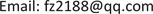Received: Sep. 26th, 2017; accepted: Oct. 7th, 2017; published: Oct. 12th, 2017ABSTRACT

The author applied Excel and Origin software to count and analyze the CET3 (College English Tests 3) scores taken by different majors (non-English majors) in TCZJ (Tourism College of Zhejiang). In order to remove accidental disturbances, this article employs the group-average formula and sum-for-years formula to analyze data to highlight the internal key factors. Taken all the analyses into consideration, a conclusion can be drawn that minor-language majors in TCZJ (Japanese, Korean, Spanish, Russian majors) have the best performance on English test among all the non-English majors from 8 departments. To some degree, it reflects that minor-language majors, with the highest average scores from every test, are most capable of adapting themselves to the changes and reform of the English test and have made the biggest progress in 2 successive tests. Through analyses, a conclusion can be drawn that the key factors to cause this phenomenon does not include areas as teachers’ qualifications, students’ language proficiency and teaching models, but are closely related to the students’ intercultural communication skills. The minor-language majors in TCZJ are required to take the intercultural communication courses. Through the course training, those students have better understanding of different cultures and better skills to communicate through different cultures. On the basis of this new-found conclusion, an efficient way to improve the proficiency of college English teaching had been figured out: improving the student’s intercultural understanding ability and their understanding of the cultural background of the target language during the process of teaching English.

Keywords:Vocational College English Teaching, CET3 Scores Gained by Non-English Majors, English Cultural Background, Intercultural Understanding Ability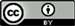1. 引言

2. 研究方法

2.1. 研究对象Table 1. The number of students participated in each CET3 test from each department during 2012-2016

2.2. 研究工具

2.3. 研究步骤

2.3.1. 使用Excel数据软件将历年历次全校各非英语专业考生CET3成绩统计分析

${\delta }_{lmn}=\stackrel{¯}{{X}_{lmn}}-\stackrel{¯}{{A}_{mn}}$ (1)

(1)式中， ${\delta }_{lmn}$ 是l系学生在m年第n次的CET3平均成绩 $\stackrel{¯}{{X}_{lmn}}$ 与m年第n次全校CET3总平均成绩 $\stackrel{¯}{{A}_{mn}}$ 的差， $\stackrel{¯}{{A}_{mn}}$ 是m年第n次CET3全校考生的平均成绩， $\stackrel{¯}{{X}_{lmn}}$ 是l系学生在m年第n次考试的平均成绩。

${\Delta }_{l}=\underset{n=1}{\overset{2}{\sum }}\left(\underset{m=12}{\overset{16}{\sum }}{\delta }_{lmn}\right)$ (2)

(2)式中， ${\Delta }_{l}$ 是l系学生从12年到16年CET3平均成绩与全校总平均成绩差值的5年总和， ${\delta }_{lmn}$ 与(1)式相同。

${\lambda }_{lm}=\stackrel{¯}{{X}_{lm2}}-\stackrel{¯}{{X}_{lm1}}$ (3)

(3)式中， ${\lambda }_{lm}$ 是l系学生在m年第二次考试与第一次考试CET3平均成绩的差， $\stackrel{¯}{{X}_{lm1}}$ 是l系学生在m年第一次考试的平均成绩， $\stackrel{¯}{{X}_{lm2}}$ 是l系学生在m年第二次考试的平均成绩。

${\Lambda }_{l}=\underset{m=12}{\overset{16}{\sum }}{\lambda }_{lm}$ (4)

(4)式中， ${\Lambda }_{l}$ 是l系学生从12年到16年第二次CET3平均成绩与第一次CET3平均成绩差 ${\lambda }_{lm}$ 的5年总和， ${\lambda }_{lm}$ 与(3)式相同，按(3)式计数获得。

${\alpha }_{mn}=\frac{\stackrel{¯}{\text{A}}}{\stackrel{¯}{{\text{A}}_{mn}}}$ (5)

(5)式中， ${\alpha }_{mn}$ 是m年第n次CET3的难度系数， $\stackrel{¯}{{A}_{mn}}$ 是m年第n次CET3全校考生的平均成绩， $\stackrel{¯}{A}$ 是从12年到16年10次全校CET3成绩的总平均，具体由(6)式求得。

$\stackrel{¯}{A}=\frac{\underset{n=1}{\overset{n=2}{\sum }}\left(\underset{m=12}{\overset{16}{\sum }}{\stackrel{¯}{A}}_{mn}\right)}{10}$ (6)

2.3.2. 比较图形制作

2.3.3. 分析成绩与专业的关系

2.3.4. 分析成绩与专业关系的关键因素

3. CET3成绩受学生主修专业影响情况分析

3.1. 不同主修专业考生历年CET3平均成绩比较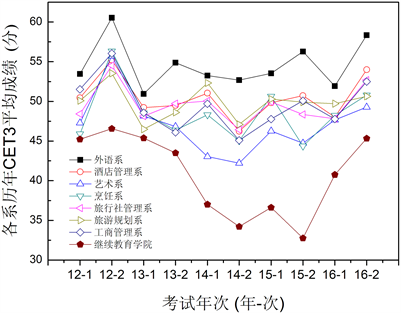Figure 1. The average CET3 scores of students from each department from 2012 to 2016

3.2. 不同主修专业考生二次CET3平均成绩提升幅度比较

3.3. 试卷难度适应能力比较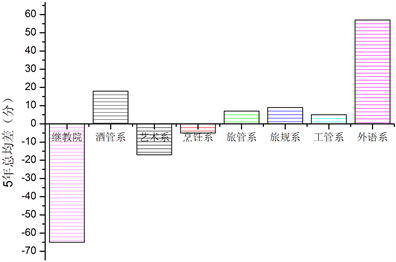Figure 2. The average deviation of CET3 Scores of each department from 2012 to 2016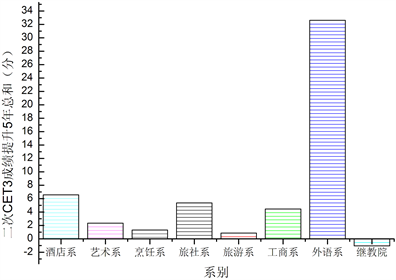Figure 3. The histogram of improvements of average CET3 scores in 2 successive tests of each department from 2012 to 2016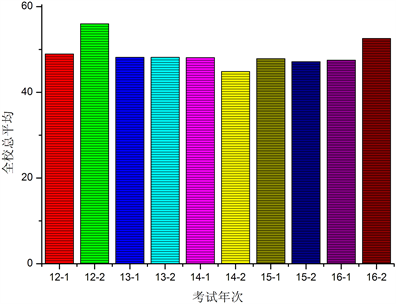Figure 4. The histogram of total CET3 average scores of each test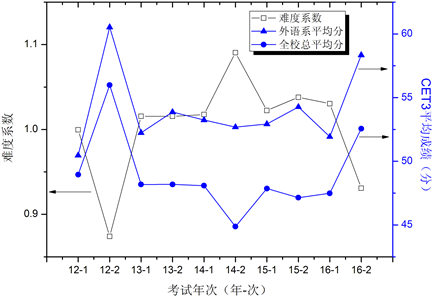Figure 5. Degree of difficulty and the average scores of CET3

4. CET3成绩受主修专业影响的关键因素分析

4.1. 新生基础不是外语系非英语专业考生CET3优势的关键因素

4.2. 师资不是外语系非英语专业考生CET3优势的关键因素

4.3. 导致外语系非英语专业考生CET3成绩明显优势的关键因素

5. 结论与建议

6. 结束语

Analyses of Key Factors to Effect CET3 Scores Taken by Different Majors in Vocational Colleges[J]. 职业教育, 2017, 06(04): 100-109. http://dx.doi.org/10.12677/VE.2017.64019

1. 1. 张媛. 高等院校大学英语教学模式探索[J]. 山东农业工程学院学报, 2016(1): 161-165.

2. 2. 张文曦. 混合式教学模式在英语写作过程教学法中的应用[J]. 现代教育科学, 2014(4): 40-43.

3. 3. 魏姝. 学生英语学习需求视角下“同课异构”模式探索[J]. 高教论坛, 2013(8): 54-57.

4. 4. 崔艳辉, 王轶. 翻转课堂及其在大学英语教学中的应用[J]. 中国电化教育, 2014(14): 116-121.

5. 5. 蔡玲. 基于项目式教学的大学生英语自主学习能力培养[J]. 现代教育科学, 2014(3): 173-175.

6. 6. 王建惠. 浅析高职教师提高学生英语学习兴趣的策略及方法[J]. 价值工程, 2012(13): 224-224.

7. 7. 程钰, 汪静. 英语教学多维评价体系的建构与实践[J]. 高等职业教育, 2015(6): 65-68.

8. 8. 陈宗胜. 基督教文化在大学英语教学中的解析[D]: [博士学位论文]. 济南: 山东大学, 2009.

9. 9. 杜宗义. 略谈中西文化的差异和交流[J]. 北京社会科学, 2013(2): 23-27.